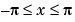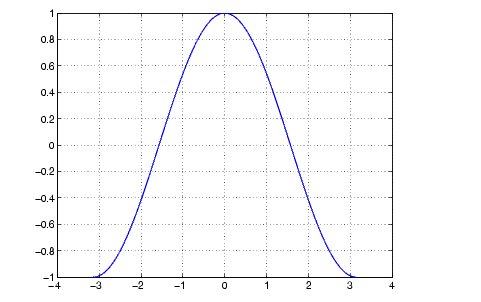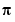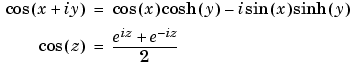MATLAB Function Referencecos

Cosine of an argument in radians

Syntax

• ```Y = cos(X)
```

Description

The `cos` function operates element-wise on arrays. The function's domains and ranges include complex values. All angles are in radians.

```Y = cos(X) ``` returns the circular cosine for each element of `X`.

Examples

Graph the cosine function over the domain.

• ```x = -pi:0.01:pi;
plot(x,cos(x)), grid on```

The expression `cos(pi/2)` is not exactly zero but a value the size of the floating-point accuracy, `eps`, because `pi` is only a floating-point approximation to the exact value of.

Definition

The cosine can be defined as

•Algorithm

`cos` uses FDLIBM, which was developed at SunSoft, a Sun Microsystems, Inc. business, by Kwok C. Ng, and others. For information about FDLIBM, see http://www.netlib.org.

See Also

`acos`, `acosh`, `cosd`, `cosh`

© 1994-2005 The MathWorks, Inc.